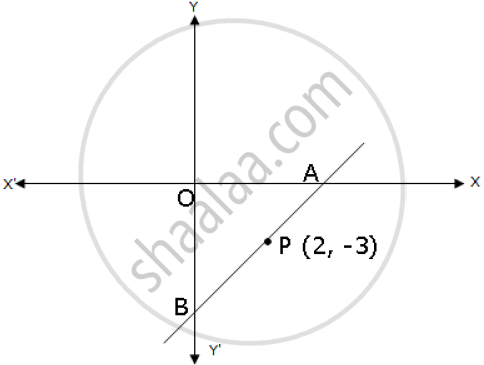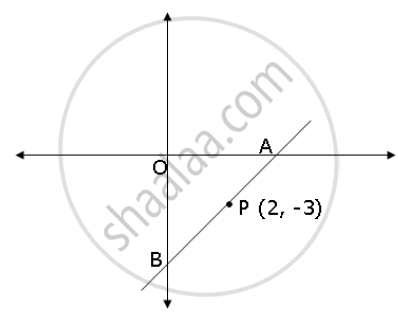Share

A and B Are Two Points on the X-axis and Y-axis Respectively. P (2, −3) is the Midpoint of Ab. Find The: (1) Coordinates of a and B (2) Slope of Line Ab. (3) an Equation of Line Ab. - ICSE Class 10 - Mathematics

Question

A and B are two points on the x-axis and y-axis respectively. P (2, −3) is the midpoint of AB. Find the:

(1) coordinates of A and B
(2) slope of line AB.
(3) an equation of line AB.Solution

1) Let the coordinates be A(x, 0) and B(0, y).

Mid-point of A and B is given by ((x+0)/2 , (y + 0)/2) = (x/2, y/2)

=> (2, -3) = (x/2, y/2)

=> x/2 = 2 and y/2 = -3

=> x =  4 and y = 6

∴ A(4,0) and B(0,-6)

2) Slope of line AB, m = (y_2 - y_1)/(x_2 - x_1) = (-6-0)/(0-4) = 3/2

3) Equation of line AB, using A(4,0)

y - 0 = 3/2 (x - 4) => 3x - 2y = 12Is there an error in this question or solution?

APPEARS IN

2009-2010 (March) (with solutions)
Question 2.3 | 4.00 marks

Video TutorialsVIEW ALL 

Solution A and B Are Two Points on the X-axis and Y-axis Respectively. P (2, −3) is the Midpoint of Ab. Find The: (1) Coordinates of a and B (2) Slope of Line Ab. (3) an Equation of Line Ab. Concept: Slope of a Line.
S